### 9.5.1.2 Axioms of rationality

To meet the goal of designing a utility function, it turns out that the preferences must follow rules called the axioms of rationality. They are sensible statements of consistency among the preferences. As long as these are followed, then a utility function is guaranteed to exist (detailed arguments appear in [268,831]). The decision maker is considered rational if the following axioms are followed when defining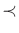and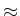:9.6

1. If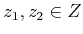, then either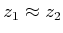or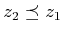.
You must be able to make up your mind.''

2. Ifand, then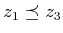.
Preferences must be transitive.''

3. If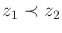, then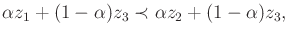(9.84)

for any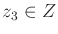and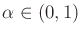.
Evenly blending in a new distribution does not alter preference.''

4. If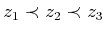, then there exists someand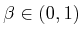such that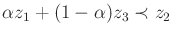(9.85)

and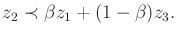(9.86)

There is no heaven or hell.''

Each axiom has an intuitive interpretation that makes practical sense. The first one simply indicates that the preference direction can always be inferred for a pair of distributions. The second axiom indicates that preferences must be transitive.9.7 The last two axioms are somewhat more complicated. In the third axiom,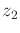is strictly preferred to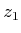. An attempt is made to cause confusion by blending in a third distribution,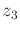. If the same amount'' ofis blended into bothand, then the preference should not be affected. The final axiom involves,, and, each of which is strictly better than its predecessor. The first equation, (9.85), indicates that ifis strictly better than, then a tiny amount ofcan be blended into, withremaining preferable. Ifhad been like heaven'' (i.e., infinite reward), then this would not be possible. Similarly, (9.86) indicates that a tiny amount ofcan be blended into, and the result remains better than. This means thatcannot be hell,'' which would have infinite negative reward.9.8

Steven M LaValle 2020-08-14